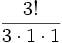# Hook-length formula

(Redirected from Hook length formula)

## Statement

Suppose$\lambda$ is an unordered integer partition of a natural number$n$. We can describe$\lambda$ by means of a Young diagram (also called a Ferrers diagram).

For every box in this Young diagram, define the hook-length at that box as follows:

Hook-length = 1 + (number of boxes directly below it vertically) + (number of boxes directly to the right of it horizontally)

Then, we have the following:

Degree of irreducible representation corresponding to partition$\lambda$ = Number of (standard) Young tableaux with shape$\lambda$ = Number of paths in the Young lattice from the trivial partition of$1$ to$\lambda$ =$\frac{n!}{\prod \operatorname{hook-lengths}}$

The product in the denominator is the product, over all boxes in the Young diagram, of the hook-length corresponding to that box.

## Particular cases$n$$\lambda$ Young diagram Hook lengths Hook-length formula Result of formula Cross-check
1 1 PLACEHOLDER FOR INFORMATION TO BE FILLED IN: [SHOW MORE] 1 (for unique box)$\frac{1!}{1}$ 1 unique Young tableau: fill box with$1$
2 22 (for left box), 1 (for right box)$\frac{2!}{2 \cdot 1}$ 1 unique Young tableau: 1 in left box, 2 in right box
2 1 + 12 (for top box), 1 (for bottom box)$\frac{2!}{2 \cdot 1}$ 1 unique Young tableau: 1 in top box, 2 in bottom box
3 33 (for left box), 2 (for middle box), 1 (for right box)$\frac{3!}{3 \cdot 2 \cdot 1}$ 1 unique Young tableau: 1 in left, 2 in middle, 3 in right box
3 1 + 1 + 13 (for top box), 2 (for middle box), 1 (for right box)$\frac{3!}{3 \cdot 2 \cdot 1}$ 1 unique Young tableau: 1 in top, 2 in middle, 3 in bottom box
3 2 + 13 (for top left box), 1 (for right box), 1 (for bottom box)$\frac{3!}{3 \cdot 1 \cdot 1}$ 2 Two possibilities for the Young tableau:
1 in top left, 2 in right, 3 in bottom
1 in top left, 3 in right, 2 in bottom
4 44,3,2,1 as we move from left-most to right-most box$\frac{4!}{4 \cdot 3 \cdot 2 \cdot 1}$ 1 unique Young tableau: 1,2,3,4 filled left to right